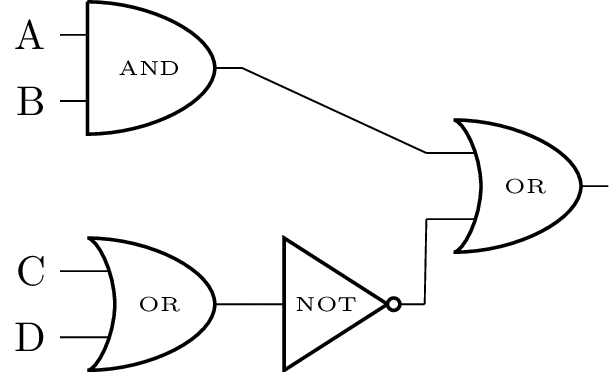시간 제한 메모리 제한 제출 정답 맞은 사람 정답 비율
1 초 (추가 시간 없음) 512 MB 15 13 12 85.714%

## 문제

You are enrolled in the Computer Organization and Architecture course at your university. You decide to write a program to help check your work by computing the output value of a combinational digital circuit, given its inputs.Figure A.1: An example of a combinational digital circuit. See the text for an explanation.

Consider the circuit shown in Figure A.1, which we use for illustration. This circuit has four inputs (letters A through D on the left), each of which is either true or false. There are four ‘gates’ each of which is one of three types: AND, OR, or NOT. Each gate produces either a true or false value, depending on its inputs. The last gate (the OR on the right) produces the output of the entire circuit. We can write these three types of gates in text by their equivalent logical operators: * for AND, + for OR, and - for NOT. In what follows, we’ll use the operators rather than gates to describe circuits.

Here is how these operators work. Given an assignment of true (T) or false (F) for each input, the operators produce the truth value indicated in the following tables:

A B A B * A B +
T T T T
F T F T
T F F T
F F F F
A A -
T F
F T

Notice that AND and OR take two inputs, whereas NOT operates on only one input. Also, we use postfix notation to write expressions involving operators (like A B *), where the operator comes after its input(s) (just as how in Figure A.1, each gate in the circuit diagram comes after its inputs).

When we describe a valid circuit in postfix notation, we use the following syntax.

• An uppercase letter (A through Z) is a valid circuit. In other words, an input alone (without any gates) is a valid circuit (which produces as output its own input value).
• If <C1> and <C2> are valid circuits, then ‘<C1> <C2> *’ is a valid circuit that produces the AND of the outputs of the two subcircuits.
• If <C1> and <C2> are valid circuits, then ‘<C1> <C2> +’ is a valid circuit that produces the OR of the outputs of the two subcircuits.
• If <C1> is a valid circuit, then ‘<C1> -’ is a valid circuit that produces the NOT of <C1>’s output.

No other description is a valid circuit.

Thus, one of the ways the circuit in Figure A.1 could be described using postfix notation is as the string:

A B * C D + - +

Given a truth value (T or F) for each of the inputs (A, B, C, and D in this example), their values propagate through the gates of the circuit, and the truth value produced by the last gate is the output of the circuit. For example, when the above circuit is given inputs A=T, B=F, C=T, D=F, the output of the circuit is F.

Given an assignment to variables and a circuit description, your software should print the output of the circuit.

## 입력

The first line of the input consists of a single integer n, satisfying 1 ≤ n ≤ 26, denoting the number of input variables. Then follows a line with n space-separated characters. Each character is either T or F, with the ith such character indicating the truth value of the input that is labeled with the ith letter of the alphabet.

The last line of input contains a circuit description, which obeys the syntax described above. Each circuit is valid, uses only the first n letters of the alphabet as input labels, and contains at least 1 and at most 250 total non-space characters.

Note that while each variable is provided only one truth value, a variable may appear multiple times in the circuit description and serve as input to more than one gate.

## 출력

Print a single character, the output of the circuit (either T or F), when evaluated using the given input values.

## 예제 입력 1

4
T F T F
A B * C D + - +


## 예제 출력 1

F